# How to specify Decimal Precision and scale number in MySQL database using PHPMyAdmin?

You need to select a database when you are creating a table. Right now, I have a sample database. The snapshot is as follows: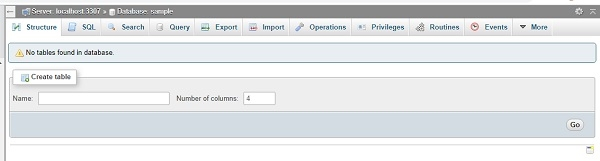Now you need to give the table name as well as the number of columns you want: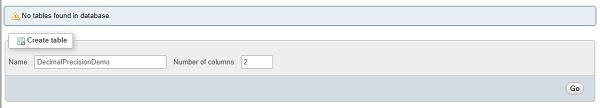After that you need to press Go button. Now, the following section would be visible: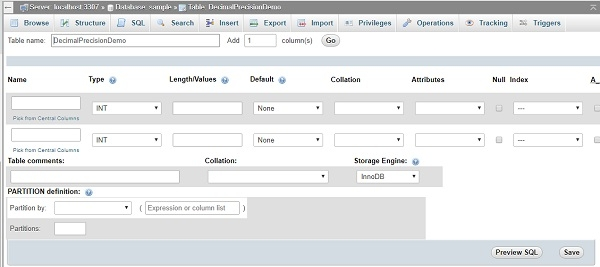The DECIMAL requires two parameter i.e. Total Number of Digit and second one is DigitAfterDecimalPoint.

The structure of DECIMAL is as follows:

DECIMAL(X,Y)

Here, X is TotalNumberOfDigit and Y is DigitAfterDecimalPoint.

Let us see an example:

DECIMAL(6,4)

Above, we will be having 6 digit sand 2 digit safter decimal point. For example, the valid range can be 24.5678 or 560.23 etc.

Here is the setup in phpmyAdmin. The snapshot is as follows: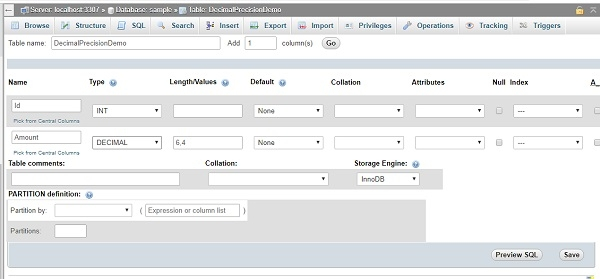After giving column name for a table, you need to use “Save” button. Here is the structure of table: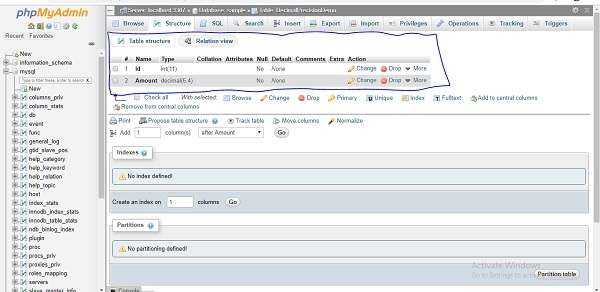There are two columns in the table DecimalPrecisionDemo. One is Id which is of type int and second one is Amount of type DECIMAL(6,4).